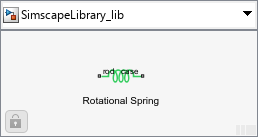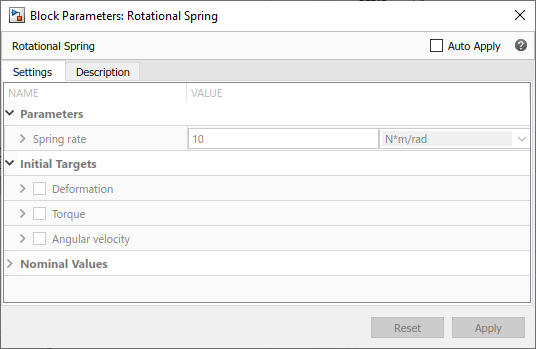## Customize Block Display

This example shows a complete component file with annotation and the resulting library block and dialog box. The image file, `custom_spring.jpg`, is located in the same folder as the component file. This example is an illustration of basic techniques described in Customizing the Block Name and Appearance.

```component spring % Rotational Spring % This block implements a simple rotational spring. nodes r = foundation.mechanical.rotational.rotational; % rod c = foundation.mechanical.rotational.rotational; % case end annotations r : Side = left; c : Side = right; Icon = 'custom_spring.jpg'; end parameters k = { 10, 'N*m/rad' }; % Spring rate end variables theta = { 0, 'rad' }; % Deformation t = { 0, 'N*m' }; % Torque w = { 0, 'rad/s' }; % Angular velocity end branches t : r.t -> c.t; end equations assert(k>0) w == r.w - c.w; t == k * theta; w == theta.der; end end ```